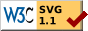Two Diagrams Illustrating the Isomorphism Extension Theorem

Two weeks ago, I had proved that any two algebraic closures of a field are isomorphic to each other in a homework problem. To finish this problem, I opened my note book to view the diagram for the Isomorphism Extension Theorem (IET) drawn before I had understood the proof of the existence of algebraic closure.1Drag the figure to translate it, and scroll to enlarge/reduce it.2
Source code: $\rm \LaTeX$, SVG

After I had read E. Artin’s construction of an algebraic closure of a field, I had also read the proof of IET.3 After that, I thought I understood this theorem. However, I couldn’t figure out how to make use of the above diagram to do this question.

\begin{equation*}\begin{CD} @. \overline{F'}\\ @. @AAA\\ E @>\tau>\cong> \tau[E]\\ @AAA @AAA\\ F @>\sigma>\cong> F' \end{CD}\end{equation*}

I can’t add dashed arrow for $\tau$.

I then opened John B. Fraleigh’s A First Course in Abstract Algebra and saw two diagrams which illustrated the IET. In those two figures, there’re only vertical and horizontal lines, no oblique lines were found.

Using these diagrams, I successfully answered this question by drawing a tower of five levels of algebraic extensions.

1. When it comes to drawing commutative diagrams, MathJax only supports AMScd, which doesn’t support diagonal arrows. Therefore, I used tikz-cd according to the last sentence of Guide to Commutative Diagram Packages by J.S. Milne to produce a standalone diagram in PDF format first. Then I converted it to an SVG file using the procedures described in the last paragraph in my earlier post about pdf2svg on Blog 1. Finally, I added the dragging and scrolling features to the SVG files after re-reading Zooming SVG in Web Browsers on Blog 1.

2. Same as footnote 1.